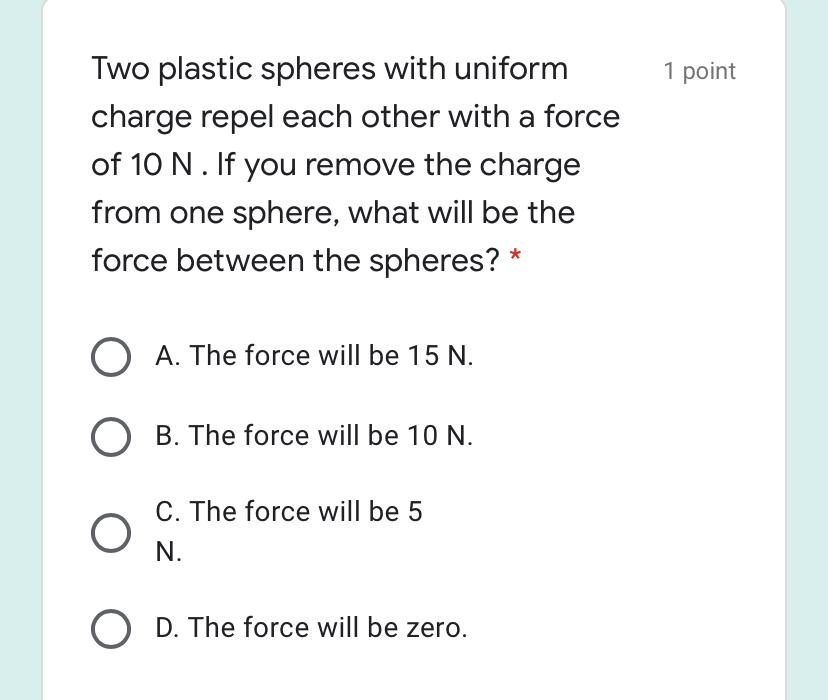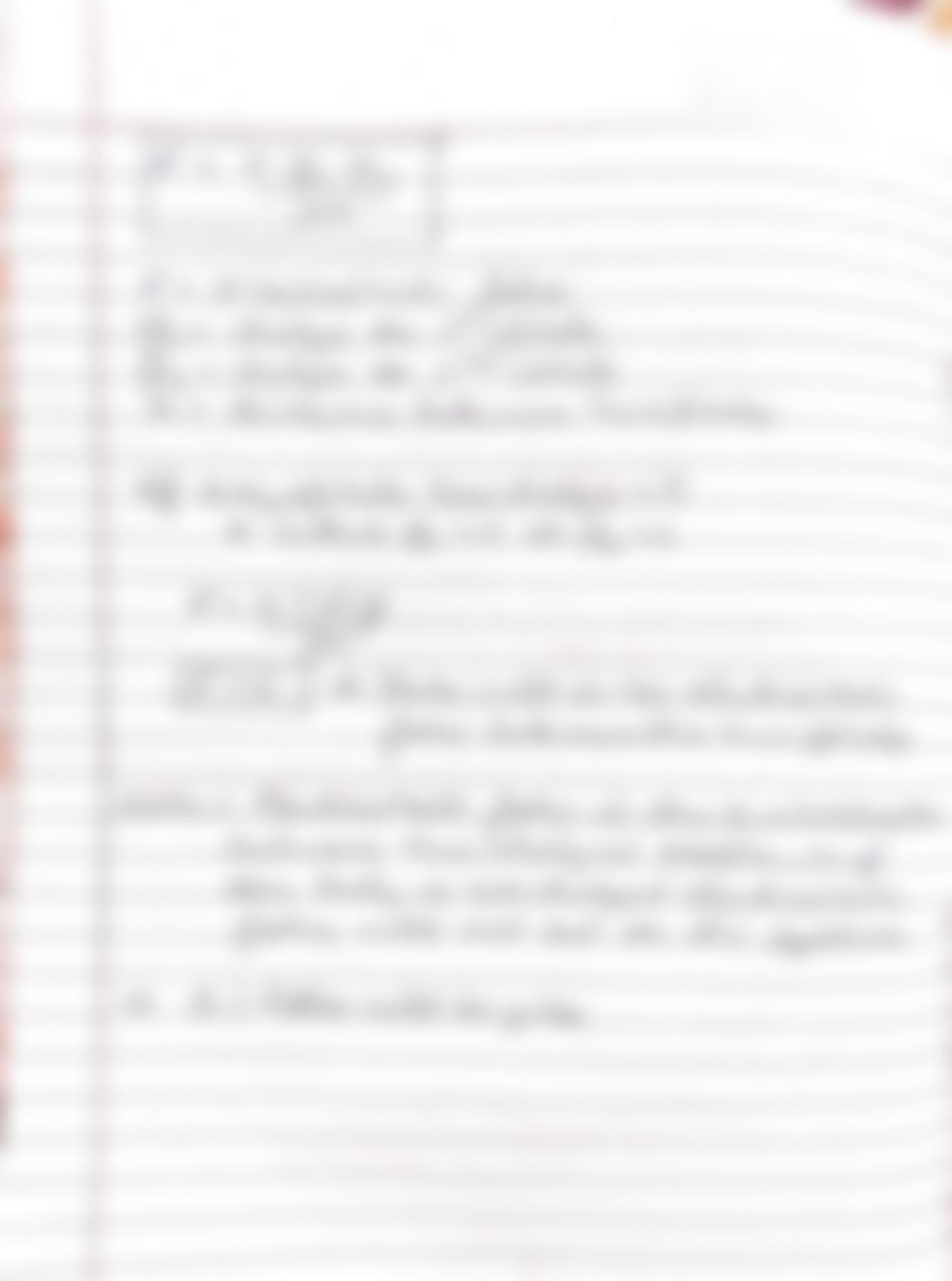Question:

1 point Two plastic spheres with uniform charge repel each other with a force of 10 N. If you remove the charge from one sphere,1 point Two plastic spheres with uniform charge repel each other with a force of 10 N. If you remove the charge from one sphere, what will be the force between the spheres? O A. The force will be 15 N. O B. The force will be 10 N. C. The force will be 5 N. O D. The force will be zero.